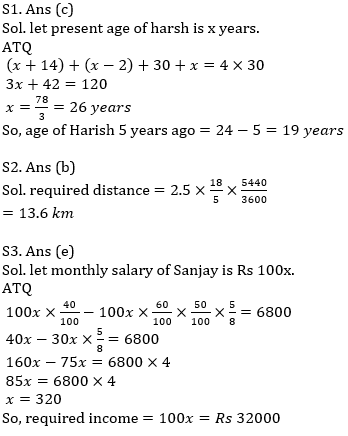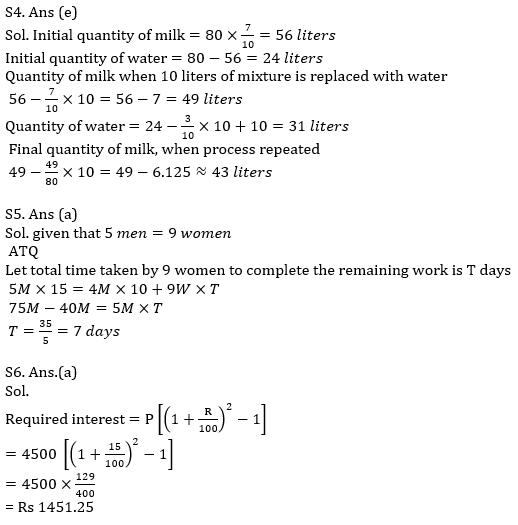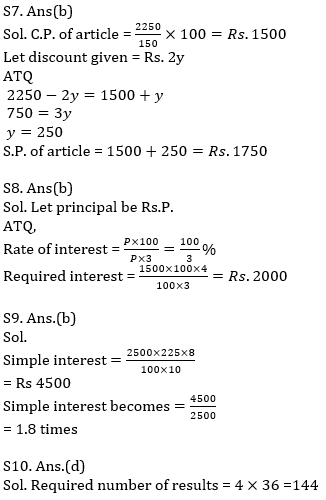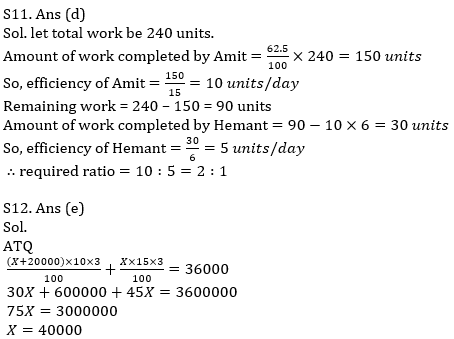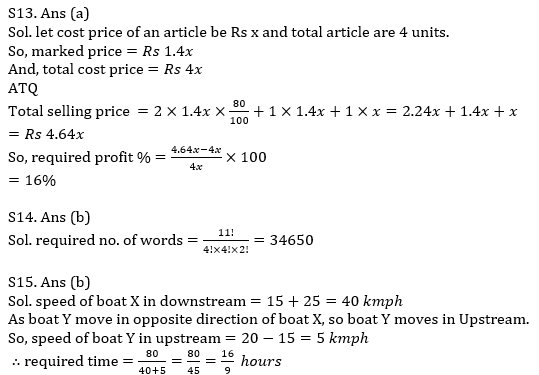Latest Banking jobs   »   Quantitative Aptitude Quiz For IBPS Clerk/NIACL...

# Quantitative Aptitude Quiz For IBPS Clerk/NIACL AO Prelims 2021- 27th August

Q1. Average of present age of Dharam, Bhavya, Harish and Harsh is 30 years. Bhavya is 14 years older than Harsh and Harish is 2 years younger than Harsh. Present age of Dharam is equal to average of present age of group. Find the age of Harish 5 years ago.
(a) 24 years
(b) 29 years
(c) 19 years
(d) 21 years
(e) 31 years

Q2. A person covers a distance with the speed of 2.5 m/sec in 1 hr 30 min and 40 sec. find distance covered by him.
(a) 12.6 km
(b) 13.6 km
(c) 15.4 km
(d) 11.8 km
(e) 14.4 km

Q3. Sanjay gives 40% of his monthly salary to his father. 50% of his remaining salary, he invests in share market and insurance in the ratio of 5 :3. If the difference between the amount he gave to his father and the amount he spent on share market is Rs 6800. Find monthly income of Sanjay.
(a) Rs 64000
(b) Rs 56000
(c) Rs 68000
(d) Rs 48000
(e) Rs 32000

Q4. A container contains 80 lit of mixture of milk and water in the ratio of 7 : 3. 10 liters of mixture is taken out from it and completely replaced by water. This process repeated again. Find the final quantity of milk in the mixture (approximate).
(a) 47 liters
(b) 40 liters
(c) 39 liters
(d) 45 liters
(e) 43 liters

Q5. 5 men or 9 women can do a piece of work in 15 days. 4 men start doing the same work and after 10 days they are replaced by 9 women. Find total time taken by 9 women to complete the remaining work.
(a) 7 days
(b) 12 days
(c) 17 days
(d) 15 days
(e) 5 days

Q6. What would be the compound interest obtained on an amount of Rs 4500 at the rate of 15% p.a. compounding annually in 2 yrs ?
(a) Rs 1451.25
(b) Rs 1144.8
(c) Rs 1482.25
(d) Rs 7750.50
(e) None of these

Q7. If M.R.P. of an article is marked 50% above cost price and profit earned on article is equal to half of discount given on article. Find S.P., if M.R.P. is Rs. 2250?
(a) Rs. 2000
(b) Rs. 1750
(c) Rs. 1850
(d) Rs. 1650
(e) None of these.

Q8. If a certain sum become twice in 3 years at certain rate at SI, then find simple interest earned on Rs. 1500 after 4 year at same rate of interest?
(a) Rs. 1500
(b) Rs. 2000
(c) Rs. 2250
(d) Rs. 1550
(e) Rs. 1750

Q9. Simple interest become what time of principle of Rs 2500 after 8 yrs at the rate of 22.5% per annum at SI?
(a) 1.2 times
(b) 1.8 times
(c) 1.5 times
(d) 2.2 times
(e) None of these

Q10. Find total no. of result that can be obtained when 2 coins are tossed and two dice are rolled simultaneously?
(a) 124
(b) 24
(c) 180
(d) 144
(e) 120

Q11. Amit starts doing a work and after 15 days of continuous work he completed 62.5% of total work. If the remaining work is completed by Amit with the help of Hemant in 6 days, find the ratio between the efficiency of Amit and Hemant.
(a) 1 :2
(b) 1 :3
(c) 2 :3
(d) 2 :1
(e) 3 :1

Q12. A person invests his money in two schemes. He invested Rs (X+20000) in scheme A at 10% SI for 3 years and Rs X in scheme B at 15% SI for 3 years. If after 3 years he got total Rs 36000 interest. Find value of X.
(a) Rs 25000
(b) Rs 35000
(c) Rs 50000
(d) Rs 45000
(e) Rs 40000

Q13. A person marks his goods 40% above the cost price. He sold half of his goods at a discount of 20%, one-fourth of the goods at marked price and the remaining at cost price. Find profit percentage.
(a) 16%
(b) 24%
(c) 12%
(d) 32%
(e) 26%

Q14. How many words can be formed using the word MISSISSIPPI.
(a) 54300
(b) 34650
(c) 12640
(d) 33640
(e) 13600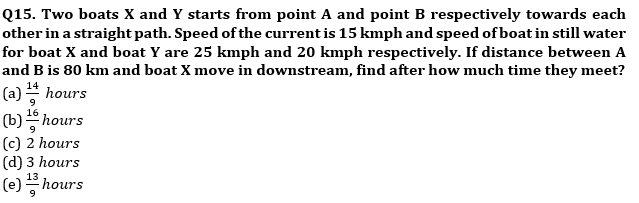Solutions